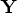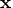Previous Page | Next Page

 Language Reference

## RANK Function

ranks elements of a matrix

RANK( matrix)

where matrix is a numeric matrix or literal.

The RANK function creates a new matrix containing elements that are the ranks of the corresponding elements of matrix. The ranks of tied values are assigned arbitrarily rather than averaged. (See the description of the RANKTIE function.)

For example, the following statements produce the vector, as shown:

```
x={2 2 1 0 5};
y=rank(x);
```

```
Y

3         4         2         1         5
```
The RANK function can be used to sort a vector, as follows:
```
b=x;
x[,rank(x)]=b;

X

0         1         2         2         5
```
You can also sort a matrix by using the SORT subroutine.

The RANK function can also be used to find anti-ranks of, as follows:

```
r=rank(x);
i=r;
i[,r]=1:ncol(x);

I

4         3         1         2         5
```

While the RANK function only ranks the elements of numerical matrices, you can rank the elements of a character matrix by using the UNIQUE function, as demonstrated in the following code:

```
/* Create RANK-like functionality for character matrices */
start rankc( x );
/* the unique function returns a sorted list */
s = unique( x );
idx = j(nrow(x), ncol(x));
ctr = 1;  /* there can be duplicate values in x */
do i = 1 to ncol( s ); /* for each unique value */
t = loc( x = s[i] );
nDups = ncol( t );
idx[ t ] = ctr : ctr+nDups-1;
ctr = ctr + nDups;
end;
return ( idx );
finish;

/* call the RANKC module */
x = { every good boy does fine and good and well every day};
r = rankc( x );
sortedx=x;
sortedx[ r ] = x;
print r, x, sortedx;

/* note that ranking is in ASCII order, where capital
letters precede lower case letters.  To get case-insensitive
behavior, transform matrix before comparison */
x = {'a' 'b' 'X' 'Y' };
asciiOrder = rankc( x );
alphaOrder = rankc( upcase( x ) );
print x, asciiOrder, alphaOrder;
```

IML does not have a function that directly computes the rank of a matrix. You can use the following technique to compute the rank of matrix A:

```
rank=round(trace(ginv(a)*a));
```Previous Page | Next Page | Top of Page